Maths-
General
Easy

Question

# If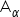=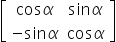, then which of following statement is true -

## The correct answer is:.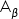=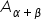and()n =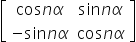### we are given matrix A and we are asked to find which of the given statements are true.By using the properties of a matrix we verify that.=and()n =Therefore the correct option is choice 4#### With Turito Foundation.#### Get an Expert Advice From Turito.